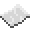# math.sqrt

math.sqrt
Function
Syntax
math.sqrt(
• num : number
)

Returns number
API math
Source Lua (source)

Returns the square root of num.ExamplePrint the square root of 2.
Prints an approximation of ${\sqrt {2}}$.
Code
<nowiki>
print(math.sqrt(2))
</nowiki>

Output 1.4142135623731